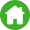Home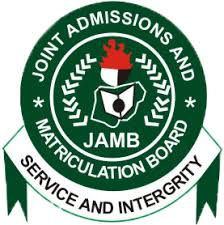Jamb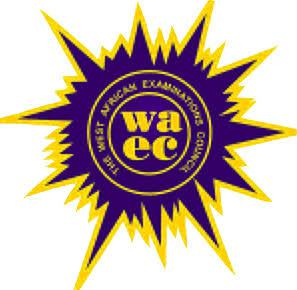Waec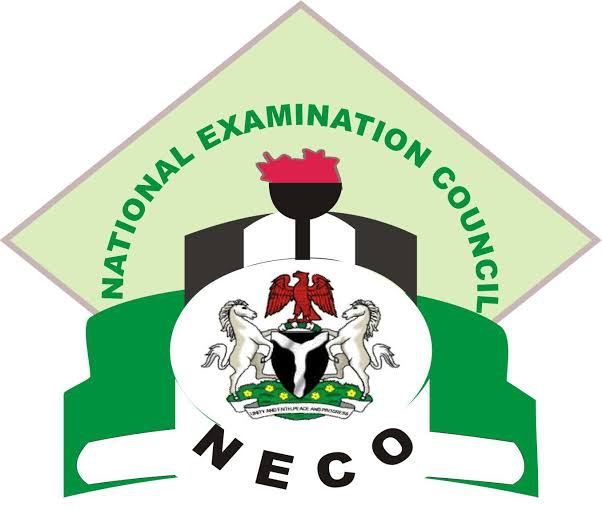Neco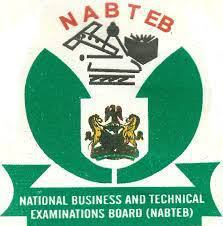NabtebNews

### SUBSCRIBE HERE!!

Quick Contact08162563540

--WAEC GCE 2019 TIMETABLE
--WAEC GCE 2019 SPECIMENS/PRACTICALSCLICK HERE FOR OUR NEW ANSWER LINK

# WAEC 2019/2020 Physics Syllabus Questions and Answers

So many waec candidates have been asking questions about 2019 physics waec syllabus and topics to read so as to pass waec Physics 2019 without much stress.

The truth of the matter is that, the relevance of Jamb syllabus and expo on the topics to focus on cannot be overemphasised. There are four weapons you need you need to pass the WAEC 2019/2020 examination. They are:

WAEC Syllabus
Hot topics to read to pass waec 2019
The recommended waec textbooks and

In this article, I will break down the waec physcis syllabus for you.

WEST AFRICAN SENIOR SCHOOL CERTIFICATE EXAMINATION WAEC PHYSICS SYLLABUS

383

SECTION A
FOR CANDIDATES IN ALL MEMBER COUNTRIES
PART I
MATTER, POSITION, MOTION AND TIME

TOPICS

NOTES

1. Concepts of matter

2. Fundamental and derived quantities and
units

(a) Fundamental quantities and units

(b) Derived quantities and unit

3. Position, distance and displacement.

(a) Concept of position as a location of
point – rectangular coordinates.

(b) Measurement of distance

(c) Concept of direction as a way of
locating a point – bearing

(d) Distinction between distance and
displacement

Simple structure of matter should be discussed.
The three states of matter, namely solid, liquid
and gas. Evidence of the particle nature of
matter e.g. Brownian motion experiment,
Kinetic theory of matter. Use of the theory to
explain: states of matter (solid, liquid and gas),
pressure in a gas, evaporation and boiling;
amorphous substances to be compared
(Arrangement of atoms in crystalline structure
not required.)

Length, mass, and time as examples of
fundamental quantities and m, kg and s as their
respective units.

Volume, density and speed as derived quantities
and m3, kgm-3 and ms-1 as their respective units.

Position of objects in space using the X,Y,Z
axes can be mentioned.

Use of string, metre rule, vernier callipers and
micrometer screw gauge. Degree of accuracy
should be noted. Metre (m) as unit of distance.

Use of compass and a protractor.

Graphical location and directions by axes to be
stressed.

WEST AFRICAN SENIOR SCHOOL CERTIFICATE EXAMINATION
PHYSICS SYLLABUS
384

TOPICS

NOTES

4. Mass and weight

Distinction between mass and weight

5. Time

(a) Concept of time as interval
between physical events

(b) Measurement of time

6. Fluids at rest

(a) Volume, density and relative
density

(b) Pressure in fluids

(c) Equilibrium of bodies

(i) Archmedes’ principle

(ii) Law of flotation

Use of lever balance and chemical/beam
balance to measure mass and spring balance
to measure weight.

Kilogram (kg) as unit of mass and newton (N)
as unit of weight.

The use of heart-beat, sand-clock, ticker-
timer, pendulum and stopwatch/clock.

Seconds (s) as units of time.

Experimental determination for solids and
liquids.

Concept and definition of pressure. Pascal’s
principle, application of principle to hydraulic
press and car brakes. Dependence of pressure
on the depth of a point below a liquid surface.
Atmospheric pressure. Simple barometer,
manometer, siphon, syringes and pumps,
determination of the relative density of liquids
with U-tube and Hare’s apparatus.

Identification of the forces acting on a body
partially or completely immersed in a fluid.

Use of the principle to determine the relative
densities of solids and liquids.

Establishing the conditions for a body to float
in a fluid. Applications in hydrometer,
balloons, boats, ships, submarines etc.

WEST AFRICAN SENIOR SCHOOL CERTIFICATE EXAMINATION
PHYSICS
385

TOPICS

NOTES

7. Motion

(a) Types of motion:
Random, rectilinear, translational,
rotational, circular, orbital, spin,
oscillatory

(b) Relative motion

(c) Cause of motion

(d) Types of force:

(i) Contact force

(ii) Force Field

(e) Solid friction

(f) Friction in fluids (Viscosity)

(g) Simple ideas of circular motion

Only qualitative treatment is required.
Illustration should be given for the various
types of motion.

Numerical problems on co-linear motion may
be set.

Force as cause of motion.

Push and pull

Electric and magnetic attractions and
repulsion; gravitational pull.

Frictional force between two stationary bodies
(static) and between two bodies in relative
motion (dynamic). Coefficients of limiting
of friction e.g. in locomotion, friction belt,
reduction of efficiency, wear and tear of
machines. Methods of reducing friction. Use
of ball bearings, rollers and lubrication.

Definition and effects. Simple explanation as
extension of friction in fluids. Fluid friction
and its application in lubrication should be
treated qualitatively. Terminal velocity and its
determination.

Experiments with a string tied to a stone at
one end and whirled around should be carried
out to

(i) demonstrate motion in a
vertical/horizontal circle.

WEST AFRICAN SENIOR SCHOOL CERTIFICATE EXAMINATION
PHYSICS
386

TOPICS

NOTES

8. Speed and velocity

(a) Concept of speed as change of
distance with time

(b) Concept of velocity as change of
displacement with time

(c) Uniform/non-uniform
speed/velocity

(d) Distance/displacement-time graph

9. Rectilinear acceleration

(a) Concept of acceleration as change
of velocity with time.

(b) Uniform/non-uniform acceleration

(c) Velocity-time graph,

(d) Equations of motion with constant
acceleration;
Gravitational acceleration as a
special case.

(ii) show the difference between angular
speed and velocity.

(iii) show centripetal force. Banking of
should be qualitatively discussed.

Metre per second (ms-1) as unit of
speed/velocity.

Ticker-timer or similar devices should be
used to determine speed/velocity. Definition
of velocity as ds/dt.

Determination of instantaneous speed/velocity
from distance/displacement-time graph and
by calculation.

Unit of acceleration as ms-2

Ticker timer or similar devices should be used
to determine acceleration. Definition of
acceleration as dv/dt.

Determination of acceleration and
displacement from velocity-time graph

Use of equations to solve numerical problems.